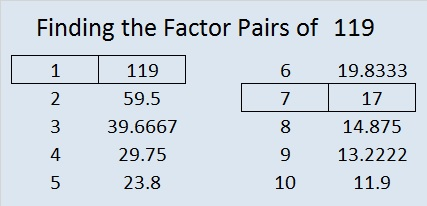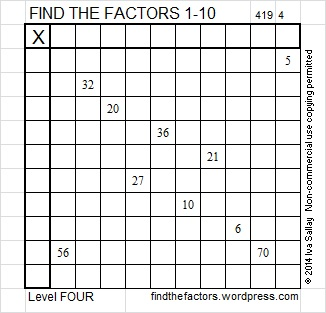# 119 and Level 4

119  is a composite number. 119 = 1 x 119 or 7 x 17. Factors of 119: 1, 7, 17, 119. Prime factorization: 119 = 7 x 17.119 is never a clue in the FIND THE FACTORS puzzles.

119 is the sum of consecutive prime numbers two ways. Each way begins with one of its prime factors!
7 + 11 + 13 + 17 + 19 + 23 + 29 = 119 (That’s 7 consecutive primes!)
17 + 19 + 23 + 29 + 31 = 119Excel file of this week’s puzzles and last week’s factors: 10 Factors 2014-05-12This site uses Akismet to reduce spam. Learn how your comment data is processed.